# Qlik Design Blog

All about product and Qlik solutions: scripting, data modeling, visual design, extensions, best practices, etc.

AnnouncementsEmployee

## The Magic of Variables

Variables can be used in many ways in QlikView. They can have static values or they can be calculated. But when are they calculated?  At script run-time or when the user clicks? And how should they be called? With or without dollar expansion?

One basic way to assign a value to a variable is to use a Let statement in the script:

Let vToday  = Num(Today()) ;

This will calculate the expression and assign it to the variable when the script is run. This is exactly what you want if you want to use a variable as a numeric parameter in your expressions.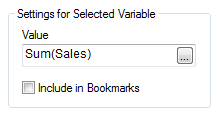But if you want the expression to be evaluated at a later stage, e.g. every time the user clicks, what should you do then? One way is to store the expression as a string in the variable, using either the Set or the Let statement or by defining it in the Document Properties -> Variables:

Set vSales  = Sum(Sales) ;

Let vSales  = 'Sum(Sales)' ;

In neither case, the expression will be calculated. The variable will contain the string ‘Sum(Sales)’, which subsequently can be used in an expression using a dollar expansion: \$(vSales).

With a dollar expansion, QlikView will substitute the ‘\$(vSales)’ with ‘Sum(Sales)’ before the expression is evaluated. Some of you will recognize this as an old style assembler macro expansion. The subsequent calculation will be made based on the evaluation of the resulting expression. Note the two steps: (1) Variable expansion; and (2) Expression evaluation.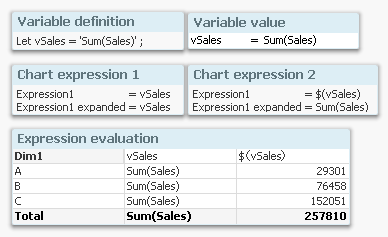In the chart above, you can see the result of using a normal variable reference (the first expression) or using a dollar expansion (the second expression). In the second expression, the variable is expanded and the numbers are calculated correctly.

But this is just the beginning…

It is also possible to calculate the variable value, i.e. determine how it should be expanded, by using an initial equals sign in the variable definition.

Let vSales2  = '=Sum(Sales)';

In this case, the variable value is calculated after each click, whereupon the dollar expansion in the chart expression is made, and finally the expression is evaluated. This means that the evaluation of ‘Sum(Sales)’ is done before the variable expansion. Note the three steps: (1) Variable calculation; (2) Variable expansion; and (3) Expression evaluation.

The table below summarizes the three methods.With the above, you can do almost magical things. You can for instance make conditional calculations that depend on e.g. selections, client platform or user.

Example:

• Create a field [Field to Aggregate] containing the names of two other numeric fields: 'Quantity' and 'Sales'
• Create a variable vConditionalAggregationField = '=Only([Field to Aggregate])'
• Create a chart with an expression = Sum(\$(vConditionalAggregationField))

The calculation in a chart will now toggle between Sum(Quantity) and Sum(Sales) depending on your selection.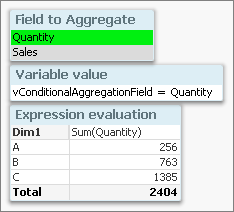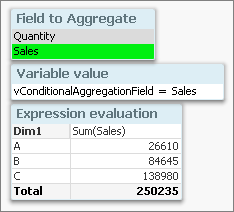The use of variables is an extremely powerful tool that you can use to create flexible applications. Use it – but with caution. Too much magic behind the curtains can be confusing.

HIC

Further reading related to this topic:

The Magic of Dollar Expansions

The Little Equals SignMaster II

Thanks Henric,

I have seen some apps where it looks like a scene from the movie Inception!

16,615 ViewsCreator II

Thanks Henric: As usual: so clear, so accurate, so essential.

CB.

16,615 ViewsPartner

Great post. Really clarifies a topic, not so well covered in any books or post until now.

I see a lot of potential with this last piece.

Thanks Henric

16,615 Views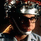Creator

Very good posting, thank you!

16,615 ViewsNot applicable

I'm printing this out and posting on my wall. Great reference!

16,615 ViewsPartner

hi HIC,

nice blog

16,615 ViewsMVP

Very straight forward and useful

12,249 ViewsNot applicable

Thank you Henrich for this good overview. I'm in the process of implementing some thing I have learned at the master summit for qlikview and one of them was about variables. I need a set analysis wherein a filter is set for every colomn of a certain table. This is the definition of the variable in the script:

SET filter='{<['&concat({<[\$Table]={"TABLE_NAME"}>}[\$Field],']=, [')&']>}';

when I use this in a text box:

sum(\$(filter) column)

It works with no problems. But when I use the same expression in a straight table I get a 0.

When I change the expression in the straight table to:

sum(\$('filter') column)

Then i get the correct behavior. How do I need to interpret this behavior in relation to your article?

Thanks

12,249 ViewsEmployee

Not sure I understand this. Can you send or post an example?

HIC

12,249 Views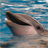Partner

Dear Henric,

I find many occasions when customers are confused as to when they can use the variable name on its own and when they need to use the \$() around the variable.  I know this forces the expansion but it does seem to me that sometimes the variable works as you want without the \$().   I also find that sometime you have to put '\$(variable)' in '' and sometimes not to get the desired result.    Do you think you could come up with a rule or rules(s) for when to use \$() or '' or is my current advice "Always put all variables used in Script or Expressions in \$() the simplest (must be) and also best option?

Thanks  Philip

12,249 Views